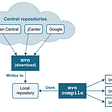# Roman To Integer (Leetcode Problem #13)

## Java Solution for https://leetcode.com/problems/roman-to-integer/

If you prefer video format : https://youtu.be/BC-mHuUAaZA

## Understanding Problem

`input_x = "LVIII"output_x = 58L = 50; V=5; III=3 `
• There some facts about roman numerals. They are usually written from largest to smallest. In above example L is larger than V.
• There some instances mentioned below where subtraction is used.
• I can be placed before (V & X); X can be placed before (L & C); C can be placed before (D & M).
• So four is not written lie IIII , rather it is written as IV.

• I can convert roman numeral string to char array.
• Since generally roman numerals are written largest to smallest usually except some cases where subtraction is needed, I can just check the case that if current char value is greater than next char value, if yes than i can just add the value of it to my sum.
• But if i have case where current roman numeral value is less than next roman value then i have to subtract it. For example if roman numeral is “IV”, in that case if my current char is I then sum = sum- Value of (I) due to subtraction rule of roman numerals
• Data structure: We need hashmap to store roman numerals and its corrosponding value
Time-complexity: O(length(N)) where N is the input roman numeral
Space-complexity: Constant Space since roman numerals are constant in time

## Pseudocode

`1: Convert Input roman numeral to char array2: Create Map of each roman numeral char and its corrosponding value3: Traverse input char array4: for each char array calculate   a: if current roman numeral value is greater than next roman      numeral  - value then add the value of current roman numeral      to the total sum    b: if the current roman numeral value is less than the next roman      numeral then we should subtract it from total sum5: finally add the last numeral value to the sum and return the total sum`

## Result

Our code beats 65% of the Java solution , not great solution but it does the job in O(N) time and O(1) space.

## Conclusion

• This problem is good excercise to understand the use case of HashMap since we need to store constant key-values and lookup the keys and corrosponding values while traversing each character in input string.
• We shouldnt hesitate to use HashMap whenever we think we need to store and access data quickly without traversing entire data structure. This typically improves such performance on added space complexity

Let me know if you have some opinion on the solution in the comment section !

Happy Leetcoding!

Liked this blog ? Find more @ : https://asyncq.com/

--

--

--

## More from Javarevisited

A humble place to learn Java and Programming better.

## Humane Debugging: Beginner’s Mind## Generating SDKs for your API## Remote access PostgreSQL in Raspberry Pi## The Kubernetes Challenge with Elton Stoneman## Using SharePoint for Master Data Management of MS Access Applications## Learn the Adapter Design Pattern## Current Location And Git Status on Mac Terminal Prompt## Suraj Mishra

Google Cloud Certified Professional Data Engineer. Find more blogs: https://asyncq.com/

## Toned-down Code Complexity Using Facade Design Pattern## Coding Algorithms — Parsing Strings## Why Build Tools Matter for a Well-Grounded Developer